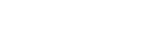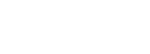# Physics Paper 1 - 2021 KCSE Prediction Questions and Answers Set 1

### Instructions to Candidates

1. This paper consists of two sections A and B
2. Answer all questions in section A and B
3. All working must be shown in the spaces provided in this booklet.
4. Mathematical tables and silent electronic calculators may be used
5. Candidates should answer the questions in English

SECTION A (25 MARKS)

Answer questions in the spaces provided.

1. The figure below shows a measuring cylinder containing some water. Another 3cm3of water was added in to the cylinder from a burette delivering volumes from 0cm3 to 50 cm 3. Record in the spaces provided the new reading indicated on each vessel.      (2 marks)1. Sketch a vernier callipers scale reading 3.41 cm.                         (1mark)
1. A uniform metallic bar of length 100cm and mass 40kg is supported horizontally by two vertical spring balances A and B as shown below.Balance A is 20cm from one end while balance B is 30cm from the other end. Find the reading of each individual balance AB.        (3 marks)
1. The reading on a mercury barometer at Mombasa is 760mm. Calculate the pressure at Mombasa
(density of mercury is 1.36x104Kgm -3)                                                                (3 marks)
1. Explain the cause of random motion of smoke particles as observed in Brownian motion experiment using a smoke cell.                                     (1 mark)
2. When a Bunsen burner is lit below a wire gauze, it is noted that the flame initially burns below the gauze as shown in the figure below.After sometime the flame burns below as well as above the gauze. Explain this observation       (2 marks)
1. The diagram below shows a flask fitted with a glass tube dipped into a beaker containing water at room temperature. The cork fixing the glass tube is tight. State with reason what would be observed if cold water is poured on to the flask.                (2marks)1. A resultant force F acts on a body of mass ‘m’ causing an acceleration of a1 on the body. When the same force acts on a body of mass 2m, it causes an acceleration of a2.Expressa2 in terms of a1. (3 marks)
2. The diagram below shows three identical springs which obey Hooke’s law.Determine the length X.  (3 marks)
1. The figure below shows a pith ball being lifted in to a funnel end of a blower.Explain this observation             (2 marks)
1. A metal ball suspended vertically with a wire is displaced through an angle as shown in the diagram below.The body is released from A and swings back to ‘B’. Given that the maximum velocity at the lowest point B is 2.5 m/s. Find the height h from which the ball is released       (3 marks)

SECTION B (55 MARKS)
Answer questions in the spaces provided.

1.
1. When is work done? (1mark)
2. The table below shows energy conversion from form A to form B and the transducers in use.
Complete the table    (5marks)
Form A                              Form B                                    Transducer
1. Chemical                             Electrical                                  __________
2. Solar                                   ______                                        Plants
3. _________________            Electrical                                  Thermocouple
4. Electrical                           Kinetic                                     __________________________
5. Electrical                            ____________________        Loudspeaker
3. When an electric pump whose efficiency is 70% raises water to a height of 15m, water is delivered at the rate of 350 litres per minute.
1. What is the power rating of the pump?                                                                    (3marks)
2. What is the energy lost by the pump per second.                                                   (3marks)
1. The figure below shows a set up that can be used to determine the specific heat capacity of a metal block.1. State the measurement that should be taken in the experiment to determine specific heat capacity of the metal block.     (3marks)
2. Show how the measurement above can be used to determine the specific heat capacity of the metal block.                           (2marks)
3. State the function of the following in the set up
1. Lagging                                                                                                                              (1mark)
2. Drops of oil in the holes containing thermometer and the electric heater                           (1mark)
4. A copper can together with stirrer of total heat capacity 600J/K contains 200g of water at 150C. Dry steam at 1000C is passed through the water while stirring until it reaches a final temperature of 550C. Calculate the mass of the steam condensed. Take specific heat of capacity of water as 4200J/Kgk and specific latent heat of steam as 2,260,000J/kg (5marks)
1.
1. A car is negotiating unbanked circular track. State two factors that will determine the critical speed of the car. (2marks)
2. Given that the car above has a mass of 1000kg and the circular path has a radius of 25m. Determine the maximum speed with which the motorist can travel so as not to skid if the frictional force between the tyres and the road is 6500N.  (3marks)
3. A 200g mass tied to a string is being whirled in a vertical circle of radius 32cm with uniform speed, At the lowest position the tension in the string is 10.5N. Calculate:-
1. The speed of the mass                                                                                             (3marks)
2. The tension in the string when the mass is at the uppermost position of the circular path (Take g = 10m/s2)          (3marks)
1.
1. State Archimedes’ principle. (1 mark)
2. The figure below shows a rectangular object of mass 100kg tethered to the sea-bed by a wire. The dimensions are 4m x 1.5m x 2m.Calculate the :-
1. Weight of sea water displaced by the buoy (density of sea water = 1100kg/m3)                             (3 marks)
2. Upward force exerted on the buoy by the water.                                                  (1mark)
3. Tension in the wire                                                                                                          (2 marks)
3. A test tube of mass 10g and uniform cross-sectional area 4cm2 is partly filled with lead shots and floats vertically in water with 5cm of its length submerged.Find the:-
1. Mass of the lead shots.(density of water = 1g/cm3)                                                                        (3 marks)
2. Length of the test tube that would be submerged in a liquid of density 0.75g/cm3.                       (2 marks)
1.
1. A car sets out from rest with constant acceleration of 0.5m/s2 for 10s. It then continues at a constant velocity for further 25s and then decelerates to rest in 5s.
1. Draw a velocity-time graph for the whole journey.                                                             (2 marks)
2. find the average speed for the whole journey.                                                                              (3 marks)
2. A body is moving eastwards at 10m/s and it decelerates at 2.0m/s2. Determine its velocity after it has traveled 24m.     (3 marks)## MARKING SCHEME

1.
1. New reading of burette = 48 cm3 (1 mark)
2. New reading of cylinder = 21 cm3     (1 mark)
1. vernier callipers2.1.1. Smoke particles are being hit by unseen air molecules moving in a random motion      (1 mark)
2. Wire gauze is a good conductor of heat and hence conduct heat away from the upper region of the wire gauze the gas reaches its ignition temperature later when the flame starts showing on the upper region.                                                                                                       (2 marks)
3.
1. Water is sucked in to the glass tube
2. Air in the flask contracts when cooled.lowering pressure inside               (2 marks)
4.5.1. High velocity air creates a low pressure inside the funnel Air outside push the ball into the low pressure area.      (2 marks)
2.1.
1. Work is done if a body moves in the direction of the force
2.
1. Cell
2. Light
3. Heat
4. Motor
5. Sound
3.
1. Power output = 0.7
Power input
350 x 15 x 10 = 0.7
60 x power input
Power input = 350 x 15 x 10
60 x 0.7
= 1250w
2. Power lost = 30  x 1250
100
= 375w
Energy lost  per s= 375J/S√
1.
1. Mass of metal block = m
Time taken to heat the block = t
Initial temperature of block = θ1
Final temperature of block = θ2
6 @ ½ mk
2. Assuming no heat to the surrounding the electrical energy supplied by the heater is equal to heat gained by the metal block
VIt = mc(θ2 – θ1
C =     vit
m(θ2 – θ1)
3. Lagging – to insulate the set – up from heat loss prevent heat loss or gain to or from the surrounding.
Drops of oil – to improve thermal contact with the heater and thermometer.
4. Let m be mass of steam
Heat gained by water  + heat gained by the can = heat lost by steam + heat lost by hot water
ΔT1 for cold water = 55 – 15 = 40k
ΔT1  for can = 55 – 15 = 40
ΔT2 for hot water = 100 – 55 = 45
MwCΔT1+ CΔT= ML + MCΔT2
0.2 x 4200 x 40 + 600Ö x 40 = M x 2260000 + M x 4200 x 45
33600 + 24000 = 2260000M + 189000M
57600 = 2449000M
M = 0.02352kg
= 23.52g
1.
1. - Radius of the circular path
- Condition of the tyres Roughness of the tyres
Any two correct
2. Frictional force provides the centripetal force
Fr = mv2
r
v =    Fr x r
m =  6500 x 25
1000
6.5 x 25
= 12.75m/s
3.
1. m = 0.2kg, r = 0.32m
At lowest point T = mv2 + mg
r
MV2 = T – mg
r
= 10.5 – 2
= 8.5
V2 = 8.5 x 0.32
0.2
V = 8.5 x 0.32
0.2
= 3.688m/s
2. At the uppermost
MV2 = T + mg
r
T = MV2 – mg
r
0.2 x (3.688)2  – 2
r
=0.2 x (3.688)– 2
0.32
= 8.50 – 2
= 6.5N

2.
1. When  an object is partially or totally submerged, it displace a fluid  whose weight is equal to the upthrust.or when a body is totally or partially immersed in a fluid it experiences and upthrust equal to the weight of the fluid displaced.
2. volume  of water displaced 6.0m3 = 6.0 m3
Mass of water= density x volume                = 6600kg
Wt of water displaced = 6600 x 10N           = 66000 N
3. From Archimedes principle.
Upward force = 66000N (upthrust)
4. U         = W + T
66000 = 1000 + T
T= 66000N- 1000N
T = 56000 N
5. Volume of water displaced      = 5 x 4 = 20cm3
Mass of displaced water= density x volume    = 20g
Mass of test tube + lead shots = 20g (law of floatation)
Mass of lead shots = 20g – 10g = 10 g
6. Mass of liquid displaced = 20g
Volume of displaced liquid = 20/0.75 = 26.667 cm3
length of submerged tube = m/v = 26.667/5 = 5.33cm.
3.
1.
1.2. Average speed = Distance = area under the graphÖ1
time                 time
0.5(25 + 40) x 5
40
162.5
40
= 4.06 m/s
2. v2 = u2 + 2as
= 102 – 2 x 2 x 24
= 100 – 96
= 4
v = 2

• ✔ To read offline at any time.
• ✔ To Print at your convenience
• ✔ Share Easily with Friends / Students